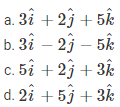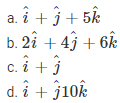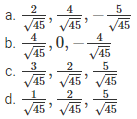# Fundamentals of Vectors MCQs for NEET

NEET  Physics is the scoring paper in the medical entrance examination. Here, you will discover the NEET Physics MCQ Questions for all Concepts as per the latest syllabus. Practice more on a regular basis with these NEET Physics objective questions on air pollution and improve your subject knowledge & problem-solving skills along with time management. NEET Physics Fundamentals of Vectors Multiple Choice Questions make you feel confident in answering the question in the exam & increases your scores to high.

## MCQ on Fundamentals of Vectors

1. The position of a particle in a rectangular coordinate system is (3,2,5). What will the position vector be?Answer: (a) $$3 \hat{i}+2 \hat{j}+5 \hat{k}$$

2. What is the displacement vector of the particle that moves from point P (2,3,5) to point Q (3,4,5)?Answer: (c) $$\hat{i}+\hat{j}$$

3. What is the vertical component of the force 5 N acting on a particle along a direction making an angle of 600?
(a) 3 N
(b) 2.5 N
(c) 10 N
(d) 4 N

4. What is the direction of cosines of vector $$\vec{A}=2 \hat{i}+4 \hat{j}-5 \hat{k} ?$$Answer: (a) $$\frac{2}{\sqrt{45}}, \frac{4}{\sqrt{45}},-\frac{5}{\sqrt{45}}$$

5. What is the minimum number of coplanar vectors with different magnitudes that can be added to get a resultant of zero?
(a) 1
(b) 2
(c) 3
(d) 4

6. A fly in the hall of dimensions 10 m×12 m×14 m starts to fly from one corner and ends up at the diametrically opposite corner. What is the magnitude of its flight?
(a) 17 m
(b) 20 m
(c) 26 m
(d) 36 m

7. Five equal forces of 10 N are applied at a point. If the angle between them is equal, what is the resultant force?
(a) 10 N
(b) 10√2N
(c) 20 N
(d) 0

8. Any vector in an arbitrary direction can be replaced by two or three vectors
(a) perpendicular to each other and have the original vector as their resultant
(b) Parallel to each other and have the original vector as their resultant
(c) Arbitrary vectors which have original vectors as their resultant
(d) It is impossible to resolve a vector

Answer: (c) Arbitrary vectors which have original vectors as their resultant

9. Angular momentum is
(a) A scalar vector
(b) An axial-vector
(c) A polar vector
(d) A displacement vector

10. Which of the following is a vector?
(a) Surface Tension
(b) Moment of Inertia
(c) Pressure
(d) None of the above

Answer: (d) None of the above

11. Which of the following is a scalar quantity?
(a) Acceleration
(b) Electric Field
(c) Work
(d) Displacement